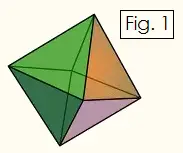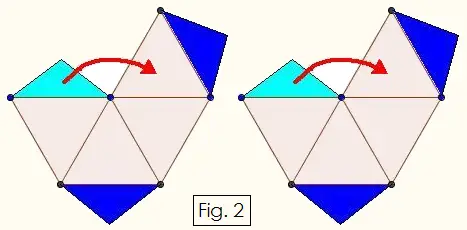# Constructing Geometric Solids:Octahedron

An octahedron is an 8-sided solid figure in which each face is an equilateral triangle. Each of the six vertices is the meeting point of four triangles. The octahedron is one of the five Platonic solids and is the dual of the cube, that is, each vertex of the octahedron corresponds to a face of the cube, and vice versa.

Octahedrons are a popular choice for 8-sided die. You can construct an octahedron using glue and stiff paper or cardstock. Just follow the instructions and pattern given below.Examine at the Figure 1 on the right to see how an octahedron is constructed from eight equilateral triangles. We will construct the octahedron by making two square pyramids and gluing them together.

Cut out the two patterns shown below in Figure 2. Crease upward along every line, and glue the light blue tab onto the adjacent triangle as shown. Also fold the dark blue tabs toward the inside of the figure, but do not glue them. Each half should resemble a hollow square pyramid with two flaps on opposite sides.Fit the two pieces of the octahedron together so that the dark blue flaps are inserted inside the figure. Secure the flaps with glue. When the glue is dry you can number the faces with the digits 1 through 8 to make a die for board games.